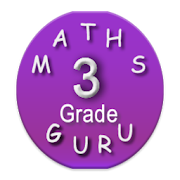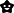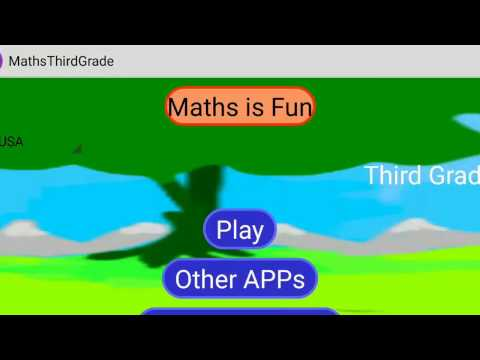Third Grade Kids Math GuruEveryoneAges 6-12
9Third grade mathematics tests (Infinite numbers - System generated questions and answers,test, questions are Different every time) for Third grade students.
This third grade learning games free contains Syllabus based on Common Core Standards. 3rd grade math games contains Levels to make your Kid Expert.

***Third grade maths APP contains
► 3.OA Represent and solve problems (multiplication and Division)
► 3.OA Word Problems
► 3.OA Multiplication games
► 3.OA Division games
► 3. NF Fractions
► 3.OA Equations (properties and relationship)
► 3.OA Multiplication & Divsion
► 3.OA all four operations (Addition, Subtraction,Multiplication & Divsion)
► 3.NBT Numbers and operations in base ten
► 3.MD Measurement
► 3.MD Time
► 3.MD Represent and interpret data
► 3.G Geometry

Third grade maths common core syllabus/ tests/ quiz/ worksheets / Third grade math activities:
Third class games / games for class third / third grade math activities: 3.OA Operations and Algebraic Thinking
► Multiplication flashcards for 3rd grade/ problems for Third grade mathematics
► Division problems for Third grade mathematics
► free multiplication and division within 100 to solve word problems
► properties of operations - Commutative property of multiplication , Associative property of multiplication , Distributive property
► arithmetic patterns (including patterns in the addition table or multiplication table)
► four operations, and identify and explain patterns in arithmetic
► Subtraction games 3rd grade

3rd grade math common core syllabus 3.NBT Number and Operations in Base Ten with Worksheets / activities
► place value for 3rd graders to understand properties of operations to perform multi-digit arithmetic in baseten
► add and subtract - place value, properties of operations, and/or the relationship between addition and subtraction in base ten
► Multiply one-digit whole numbers by multiples of 10.

Third class games / games for class third / third grade math activities: 3.NF Number and Operations Fractions
► fractions as numbers for 3rd grade students
► Test understanding of fractions
► fraction as a number on the number line
► two fractions as equivalent (equal) if they are the same size
► recognize and generate simple equivalent fractions
► Express whole numbers as fraction
► Compare two fractions-same numerator /denominator

third class games / Worksheets - third grade math activities- common core syllabus 3.MD Measurement and Data
► measurement and estimation of intervals of time, liquid volumes, and masses of objects
► time to the nearest minute and measure time intervals in minutes
► measure and estimate liquid volumes and masses of objects using standard
► geometric measurement: area and relate area to multiplication and to addition
► third grade geometry : area of a rectangle,perimeter, Multiply side lengths

third class games / Worksheets / tests / quiz / common core math games : 3.G Geometry
► Solve problems related to reasoning with shapes and their attributes
► Understand that shapes in different categories
► Partition shapes into parts with equal areas and solve problems / worksheets

With this third grade learning games free app, learn Mathematics wherever you are. 3rd grade math testing preparation helps your kid to become expert.
This kids math games for third grade is useful for school students of second grade, third grade and fourth grade to learn and test their understanding in one go.

Tag : 3rd grade learning games free, games for 8 year olds, math games for 3rd graders free , grade 3 math, free kids learning games reading math for 2nd grade and third grade, games for class third, 3rd class games, maths practice app for class 3rd, math 3rd grade, kids math 6 years old, math class 3, third grade math games free.

Collapse

Review Policy
4.4
9 total
5
4
3
2
1

What's New

Initial version
Collapse

Eligible for Family LibraryEligible if bought after 7/2/2016. Learn More
Updated
October 18, 2013
Size
2.1M
Installs
1,000+
Current Version
16.0
Requires Android
2.2 and up
Content Rating
Everyone
Permissions
Offered By
Appraiser Kids Games
Developer
42,48th Street, 9th Avenue, Ashok Nagar, Chennai, India. Postal code - 600083